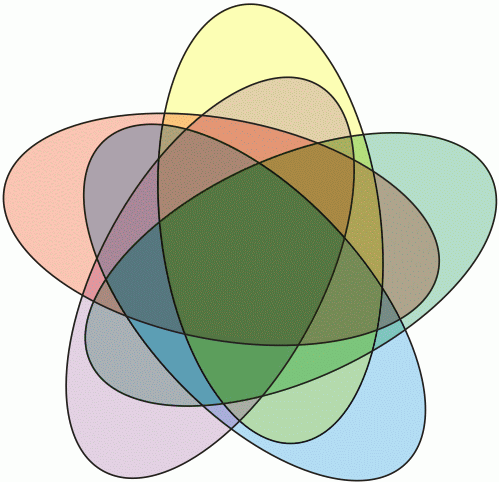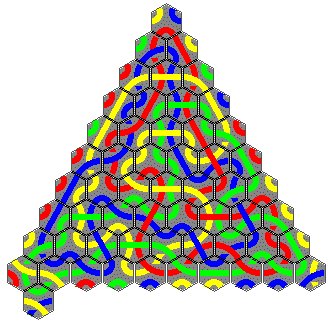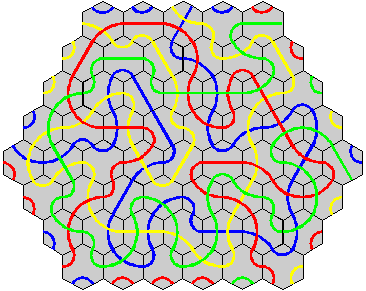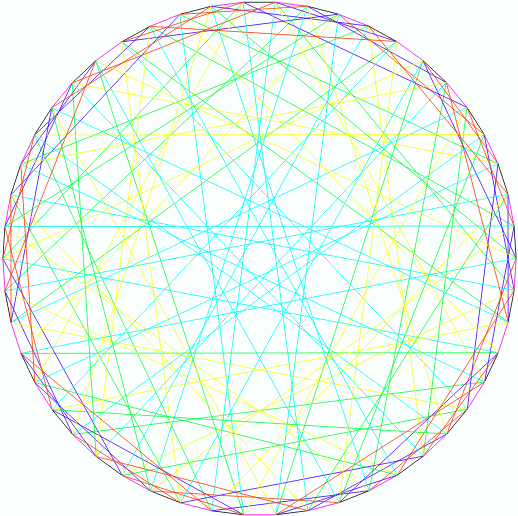## The Hoffman-Singleton Game

Ed Pegg Jr., November 1, 2004

The standard deck of cards has 13 cards in each of 4 suits. Mathematically, 4×13 = 52 cards isn't all that exciting. In most games, certain cards are better than others. Can a more interesting deck of cards be made?

The Game of Set works on 3 colors, 3 numbers, 3 shadings, and 3 symbols. Mathematically, 34 = 81 cards is nice, but may be too many cards.

A deck of double-9 domino cards has (11×10)/2 = 55 cards. Lots of nice properties. The only domino deck in print I know of is Auf&Ab, by me.

A card set based on a larger Venn diagram might be nice. I haven't really looked at the game possibilities. Here is the order-5 Venn diagram.Figure 1. An order-5 Venn diagram. Originally by Branko Grunbaum. See Frank Ruskey's survey.

Polyforms (especially hexominoes and octiamonds), make interesting sets. They don't work well as cards, though. In the various games played with Octiamonds, for example, the shapes of the pieces is more important than anything.

The edgematching sets developed in 1926 by Percy MacMahon all work well. Path cards, such as in Kaliko, Tantrix, and the 36 path dominoes also make nice games. Unfortunately for both of these, squares, hexagons, and triangles all work better as tiles.The 4 LONGEST LOOPS * red = 33  * yellow = 30  * blue = 35  * green = 38  * total length = 136  found by Milan Kuchtiak * Spisska Nova Ves * Slovakia * September 1998 The 4 LONGEST LINES * red = 34  * yellow = 37  * blue = 35  * green = 40  * total length = 146  found by Jamie Sneddon and Paul Martinsen  * Auckland * New Zealand * April 1998
Figure 2. Record-setting paths in the game of Tantrix.

In 1960, Edward F. Moore asked Alan Hoffman and Robert Singleton a question. Moore showed them that the number of vertices v in a graph had an upper bound based on the vertex degree Δ and diameter d: v ≤ 1 + Δ + Δ(Δ-1) + ... + Δ(Δ-1)d-1. He asked whether their were graphs which reached the upper bound. Hoffman and Singleton answered with the paper "On Moore Graphs with Diameter 2 and 3." They gave the start of a proof (finished in 1971) that the only possible Moore graphs were the following:

Name
Degree Δ (edges per vertex)
Diameter d
Vertices v
Pentagon
2
2
5
Heptagon
2
3
7
Petersen Graph
3
2
10
Hoffman-Singleton Graph
7
2
50
???
57
2
3250
Figure 3. The four known Moore Graphs (and maybe a fifth).

In addition, they discovered the fourth graph -- now called the Hoffman-Singleton graph -- one of the most remarkable objects in mathematics.Whether the last graph exists has been a famous unsolved problem since 1960.

There are fifty vertices in the Hoffman-Singleton graph, so we need 50 cards. Pick three numbers from {1, 2, 3, 4, 5, 6, 7}. There are 35 ways to do this, from 123 to 567. I'll call one of these cards a triad. Triplets 136, 613, 361, 631, 316, and 163 all have the same three numbers, and are thus the same triad: 136.

Fifteen more cards are Fano planes, which I will call a fano. The first fano in figure 4 contains seven triads: 136, 235, 145, 127, 347, 567, and 246 (the circle). If you select any two of these fanos, they will share exactly one triad. Each of the 35 possible triads is found in exactly 3 Fano planes.Figure 4. The fifteen Fano planes needed for the Hoffman-Singleton graph

That's enough to make the graph. Every fano is connected to 7 triads. Every triad is connected to 3 fanos and 4 triads. Every card is connected to exactly 7 other cards. For any two cards that are not connected, there is exactly 1 card connected to both. Examples: 123 and 234 are both connected to 567, 123 and 345 are both connected to the 11th fano, 123 and the last fano are both connected to 457. It's all controlled by the connection rules.

CONNECTION RULES
1. Two fanos are never connected.
2. If a fano contains a triad, they are connected.
3. Two triads with no numbers in common are connected.

Here is a PDF file of the Hoffman-Singleton deck. (I put this PDF, the deck, and the game into the public domain). If anyone makes improvements to the deck or creates nice games, please let me know.

Here is what the full graph looks like. I wanted to add the fanos and triads to the edges so that triads were connected by the color they lack, but I'm starting to think this may be impossible.Figure 5. The Hoffman-Singleton graph. Seven-coloring found by Gordon Royle.

The Hoffman-Singleton graph makes an absolutely amazing deck of cards. Here are some of the investigations you can make.Figure 6. Another construction. Connect vertex i of Pj to vertex i+jk (mod 5) in Qk. From MathWorld.

1. Find one of the 1260 pentagons in the deck. Example: 567--fano1--145--fano2--123. Put all of the cards connected to any card in the pentagon into stack P. Put the other cards in stack Q. Finally, put the original chosen pentagon into stack Q. Each stack will have 25 cards, and will each contain 5 pentagons of cards. In fact, it will be equivalent to Figure 6, with the pentagrams being stack P, and the pentagons in stack Q.
2. From your work in step 1, remove a pentagon from each stack. The remaining 40 cards are equivalent to the unique (6,5) cage graph.
3. From your work in step 2, remove a another pentagon from each stack. The remaining 30 cards are equivalent to one of the 4 (5,5) cage graphs.
4. Remove 15 unconnected cards. Example: the 15 fano planes. There are 100 ways to do this. The 35 remaining cards are equivalent to the Odd graph O4. Select any of the 15 original cards, and remove the 7 cards connected to it. The remaining 28 cards are equivalent to the Coxeter graph.
5. Pick two connected cards. Discard them, and all cards connected to them. The remaining 36 cards are equivalent to the Sylvester graph.
6. Pick a card, any card. Find 4 cards connected to it. Now find all 24 cards connected to those 4. This gives GeneralizedPetersenGraph[12,5].
7. Find a cycle of 6 cards. There is a unique set of 4 cards that will complete a Petersen graph.

So, lots and lots of properties. It's the ultimate deck of symmetric connections, has the right number of cards, and can nicely introduce some of the wonders of graph theory. It's a strongly regular graph, an integral graph with graph spectrum (-3)2122871, the unique (7,5)-cage graph, and a symmetric graph.

The Hoffman Singleton Game. Get some opponents and shuffle the deck. Start laying down the cards face-up, slowly, one at a time. At any time, a person may point out three or more connected cards. The first person to do so may pick one of those connected cards. The first person to complete a cycle of any size, wins.

There are 30 Fano planes using {1, 2, 3, 4, 5, 6, 7}. The 15 fanos currently in the deck are half of them. A possible add-on for the game would be the other 15 fanos, in a different style, for a 2-player game.

I'm sure there are many great games that can be played with this deck. For example, instead of paths of length 3, require cycles. For winning, require any non-planar graph. Let me know of any games that work well. There are many puzzles with this deck. What is the minimum number of cards needed to remove all cycles?

This is a really nice 50 card deck. I can be happy with it, mathematically.

References:

Andries E Brouwer, A M Cohen, A Neumaier, Distance Regular Graphs, Springer-Verlag, 1989. http://www.win.tue.nl/~aeb/drg/.

Andries E. Brouwer, "Descriptions of various graphs", http://www.win.tue.nl/~aeb/drg/graphs/.

Andries E. Brouwer, "Hoffman-Singleton Graph", http://www.win.tue.nl/~aeb/drg/graphs/Hoffman-Singleton.html.

Francesc Commellas, Charles deLorme, "The (Degree,Diameter) Problem for Graphs", http://www-mat.upc.es/grup_de_grafs/grafs/taula_delta_d.html.

Geoff Exoo, "The Hoffman-Singleton Graph", http://ginger.indstate.edu/ge/Graphs/index.html.

Chris Godsil, "Problems in Algebraic Combinatorics", the electronic journal of combinatorics 2 (1995). http://www.combinatorics.org/Volume_2/cover.html.

Chris Godsil, Gordon Royle. Algebraic Graph Theory, Springer-Verlag 2001.

Alan. J. Hoffman, R. R. Singleton, "On Moore Graphs with Diameters 2 and 3", IBM J of Res and Dev, Volume 4, Number 5, Page 497 (1960), http://www.research.ibm.com/journal/rd/045/ibmrd0405H.pdf.

Kadon Enterprises, "More about Edgematching", http://gamepuzzles.com/moredge.htm.

Gordon Royle, "Cages of Higher Valency", http://www.csse.uwa.edu.au/~gordon/cages/allcages.html.

Frank Ruskey. "A Survey of Venn Diagrams", http://www.combinatorics.org/Surveys/ds5/VennEJC.html.

Eric W. Weisstein. "Cage Graph, Coxeter Graph, Distance-Regular Graph, Generalized Petersen Graph, Graph Diameter, Girth, Hoffman-Singleton Graph, Integral Graph, Moore Graph, Sylvester Graph, Symmetric Graph, Vertex Degree" From MathWorld--A Wolfram Web Resource. http://mathworld.wolfram.com/.

Math Games archives.

Comments are welcome. Please send comments to Ed Pegg Jr. at ed@mathpuzzle.com.

Ed Pegg Jr. is the webmaster for mathpuzzle.com. He works at Wolfram Research, Inc. as an associate editor of MathWorld, and as administrator of the Mathematica Information Center.